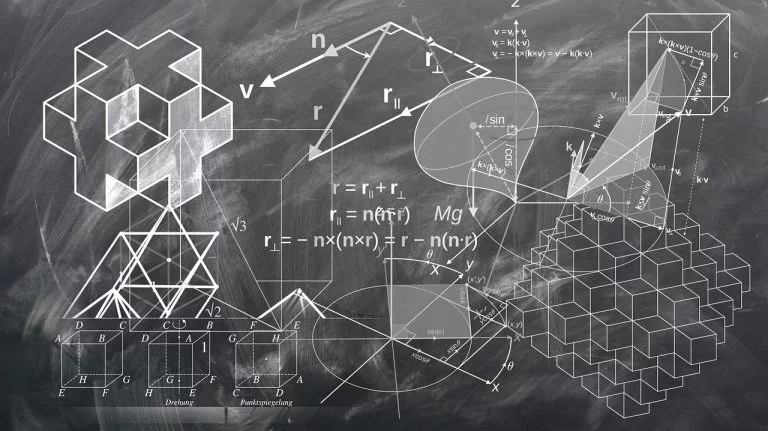# Top 10 Greatest Mathematicians Of All Time: Check Them Outgeralt / Pixabay

Mathematics has been around for thousands of years, and several mathematics geniuses have contributed to the field with their revolutionary discoveries that changed the world. It is at the core of almost every aspect of our lives including satellites, computers, medicines, astronomy, and advanced technologies. Here we are going to take a look at the top 10 greatest mathematicians of all time based on their contributions to the field.

## Top 10 greatest mathematicians

Dozens of mathematicians have made revolutionary contributions to the field of mathematics. So, it’s pretty tricky to pick the top 10 and leave many other legends out. Muhammad Al-Khowarizmi invented algebra; Aryabhatta discovered zero; John Von Neumann gave the Von Neumann architecture; and Paul Erdos published about 1,500 papers. We believe these are the top 10 greatest mathematicians.

### 10- G.F. Bernhard Riemann

Riemann was a student of the legendary mathematician Carl Friedrich Gauss. Born in 1826 in a poor family in Germany, Riemann went on to become one of the most influential mathematicians of his time. The Riemann Surfaces theory is applied in mathematical physics to this day. He made significant contributions to differential geometry and came up with various theorems including the Riemannian Geometry, Riemannian Surfaces and Riemann Integral. Many of his equations were used by Albert Einstein in the General Relativity Theory.

This Value Fund Is Well-Positioned For The COVID RecoveryDG Value Partners II, LP Class A was up 1.36% net for May, while Class C returned 2.56%. Class A has returned 18% net so far for 2021, while Class C is up 34.5% net for the first five months of the year. DG Capital touts itself as "experts in middle market event-driven investing." The Read More

### 9- Sir Isaac Newton

Newton has made significant contributions to physics and astronomy, but he was also one of the greatest mathematicians in the world. Isaac Newton is often described as the inventor of calculus. He invented calculus to explain the theory of gravitation because no other principle could explain it.

### 8- Srinivasa Ramanujan

Born in 1887 in India, Ramanujan was a self-taught mathematician. He had no formal training in mathematics. Before his death at the young age of 32, he had given more than 3,000 theorems. Ramanujan is known for his contributions to the infinite series, mathematical analysis, number theory, and continued fractions. Mathematicians are still struggling to prove many of his unsolved theorems, but almost all of his claims have been proven correct. He is widely known for Landau-Ramanujan constant, mock theta functions, Ramanujan prime, and Ramanujan-Soldner constant. Today his works are used to study blackholes.

### 7- Rene Descartes

Descartes is almost synonymous with his “Cogito Ergo Sum” philosophy. The Cartesian coordinate system was named after him. Descartes is the inventor of analytic geometry, which was used in the discovery of infinitesimal calculus and analysis. Isaac Newton and Gottfried Leibniz built upon Descartes’ work to develop modern calculus.

### 6- Gottfried Wilhelm Leibniz

Leibniz and Newton both are hailed as the inventor of calculus. But it’s Leibniz’s notation that’s used around the world today. Later mathematicians expanded his work in the infinitesimal calculus. He also restructured the binary number system that later became the foundation of modern computers. Leibniz also introduced the integral sign and invented the first fully functional electrical and mechanical calculator.

### 5- Pythagoras

Pythagoras lived between 570 and 495 BC. Thanks to the Pythagoras theorem, almost every school kid is familiar with his name. His works later influenced other great minds such as Euclid and Plato. Many consider him one of the first great mathematicians. He founded the Pythagorean cult to actively study and advance mathematics. Pythagoras theorem is used in modern measurements, though some doubt whether Pythagoras actually invented the theorem. Many claim it was invented by Baudhayana, an Indian mathematician who lived around 800 BC.

### 4- Archimedes

Born in 287 BC, Archimedes is considered one of the greatest mathematicians of all time. He laid the foundation of several crucial mathematical concepts that are at the root of modern mathematics. Archimedes anticipated modern calculus by applying the concepts of infinitesimals. He used the method of exhaustion to prove many geometrical theorems such as the area of a circle, the surface area, and the area under a parabola. He also derived an accurate approximation of Pi using the method of exhaustion.

### 3- Euclid

Euclid’s mathematical treatise Elements is still one of the most influential works in the history of mathematics. The ancient Greek mathematician was the original founder of geometry. His other works include the Euclidean algorithm, perfect numbers, and the fundamental theorem of arithmetic. He has had the greatest influence on the advancement of mathematics.

### 2- Leonhard Euler

Born in Switzerland in 1707, Euler was the most influential mathematician of the 18th century. He has made notable contributions to the graph theory, topology, analytic number theory, and infinitesimal calculus. He published more than 900 books in his lifetime. Many of his discoveries were used in solving real-world problems. Euler’s works in Latin squares form the foundation of sudoku. It was Euler who introduced the concept of a function and the Greek letter Sigma for summation.

### 1- Carl Friedrich Gauss

Born in 1777 in Germany, Gauss is often referred to as “the greatest mathematician since antiquity” and “the foremost of mathematicians.” He never published most of his work. Instead, he focused on reworking and improving his theorems. His discovery of the non-Euclidean space was found in his pile of notes after his death. Some of his most notable contributions are the Gaussian distribution, Gauss law, Gaussian optics, and Gauss-Newton algorithm. He also contributed to algebra, number theory, statistics, differential geometry, geodesy, and matrix theory.

No posts to display